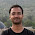MECHANICAL ENGINEERING

• SUBJECT : ENGINEERING MATHEMATICS - THEORY WITH WORKED OUT EXAMPLES SELF AND CLASSROOM PRACTICE QUESTIONS
• AUTHOR : SUBJECT EXPERTS OF ACE ENGINEERING ACADEMY - HYDERABAD
• PUBLISHERS : ACE ENGINEERING PUBLISHERS
• IDEAL FOR : GATE AND PSU's - MECHANICAL ENGINEERING

CONTENTS :
1. LINEAR ALGEBRA
2. CALCULUS
3. VECTOR CALCULUS

BRIEF INTRODUCTION TO LINEAR ALGEBRA :

INTRODUCTION : Linear algebra comprises of the theory and applications of linear system of equation,  linear transformations and eigen value problems.  In linear algebra,  we make a systematic use of matrices and to a lesser extent determinants and their properties.  Determinants were first introduced for solving linear systems and have important engineering applications in systems of differential equations,  electrical networks,  eigen value problems and so on.  Many complicated expressions occurring in electrical and mechanical systems can be elegantly simplified by expressing them in the form of determinants Cayley matrices in the year 1860.  But it was not the twentieth century was well-  discovered advanced that engineers heard of them.  These days,  however,  matrices have been found to be of great utility in many branches of applied mathematics such as algebraic​ differential equations,  mechanics theory of electrical circuits,  nuclear physics,  aerodynamics and astronomy.  With the advent of computers,  the usage of matrix methods has been facilitated.

RANK OF A MATRIX : If we select any rows and r columns from any matrix A,deleting all the other rows and columns,  then the determinant formed by these r x r elements is called the minor of A of order r.  Clearly,  there will be a number of different minors of the same order,  got by deleting different rows and columns from the same matrix.

Definition : A matrix is said to be of rank r when

(i) it has at least one non-zero minor of order r,  and
(ii) every minor of order higher than r vanishes.  Briefly,  the rank of a matrix is the largest order of any non-vanishing minor of the matrix.
If a matrix has a non-zero minor of order r,  its rank is greater than or equal to r.
If all minors of a matrix of order r + 1 are zero,  its rank is less than or equal to r
The rank of a matrix A shall be denoted by p(A).

Elementary transformation of a matrix :

The following operations,  three of which refer to rows and three to columns are known transformations L The interchange of any two rows(columns).  IT.  The multiplication of any row(column by a non-zero number.  the corresponding elements of III.  The addition of a constant multiple of the elements of any row(column to any other row(column)  Notation.  The elementary row transformations will be denoted by the following symbols:
(i) Rij for the interchange of the ith and jth rows.
(ii)  kRi for multiplication of the ith row by k.
(iii) Ri + pRj for addition to the i'th row,  p times the j'th row.  writing C in place of R

The corresponding column transformation will be denoted by matrix.  While the value of the minors may get. Elementary transformations do not change he order or rank of a changed by the transformation I and II,  their zero or non-zero character remains if one can be obtained from the(3)  Equivalent matrix.  Two matrices A and B are said to be equivalent the same other by a sequence of elementary transformations.  Two equivalent matrices have the same order and rank.  The symbol is used for equivalence

1.